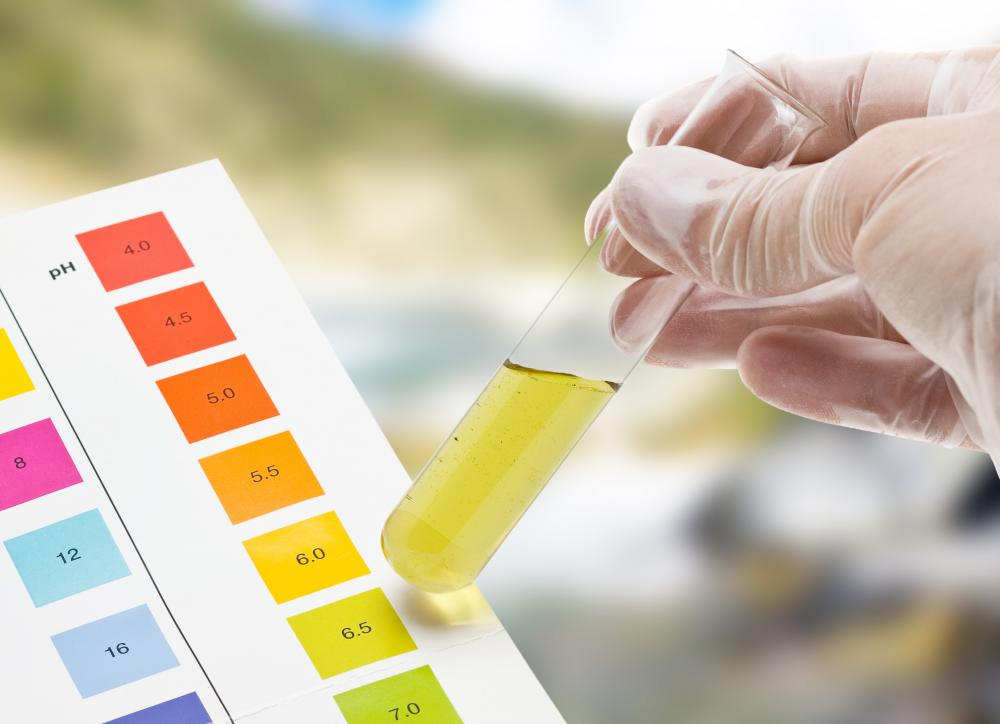# What Is the Henderson–Hasselbalch Equation?

Lynelle Harmon

The Bronsted-Lowry theory defines acids and bases based on their ability to donate, and accept, hydrogen protons. The Henderson-Hasselbalch equation builds off of those definitions to create a formula for finding the pH or concentrations of a buffer solution. Accurate buffer solutions can be used in titration or for industrial uses such as shampoo manufacturing.The Henderson-Hasselbalch equation is useful for identifying buffer solutions, which resist pH change.

According to the Bronsted-Lowry theory, an acid is any molecule capable of donating a hydrogen proton, while a base is a molecule capable of accepting the proton. Dissociation reaction of the acid, HA, results in a H+ that is donated and an A-. This A- is then called the conjugate base. The Henderson-Hasselbalch equation is built using this dissociation reaction as reworked for the acid dissociation constant.

An acid dissociation constant, denoted Ka, is the equilibrium point for the dissociation reaction. It's represented in the formula Ka = ([A-][H+]) / [HA], where A-, H+, and HA represent the respective concentrations in moles per liter. Since the Henderson-Hasselbalch equation solves for pH, the Ka equation needs to be reworked.

The conversion operates around the fact that pH = -log[H+], meaning that this should appear in the converted equation. A -log is multiplied to both sides of the Ka equation for -logKa = -log([A-][H+]) / [HA] and then logarithmic distribution converts this to -logK = -log[H+] + (-log ([A-] / [HA])). Using the definition of pH, and the fact that pKa = -logK, it can be written pKa = pH - log ([A-] / [HA]). In terms of pH, that becomes pH = pK + log ([A-] / [HA]).

The Henderson-Hasselbalch equation can be used by placing the known concentration of the conjugate base in for A- and the known weak acid concentration in for HA. An example buffer solution might include 0.10 moles acetic acid (HC2H3O2) and 0.45 moles acetate ion (C2H3O2) with a pK value of 1.7 x 10-5. The resulting equation: pH = -log (1.7 x 10-5) + log (0.45 / 0.10) = 5.4.

Solving the Henderson-Hasselbalch equation instead for the concentration of either the acid or base can determine how much to use when creating a buffer solution. Buffers are used in titrations to determine the pH level of other acid-base pairs. They also have industrial uses wherever pH needs to be well-managed. Shampoo is kept from becoming too caustic and beer kept from spoiling due to the addition of well-calculated buffers.

## You might also Like